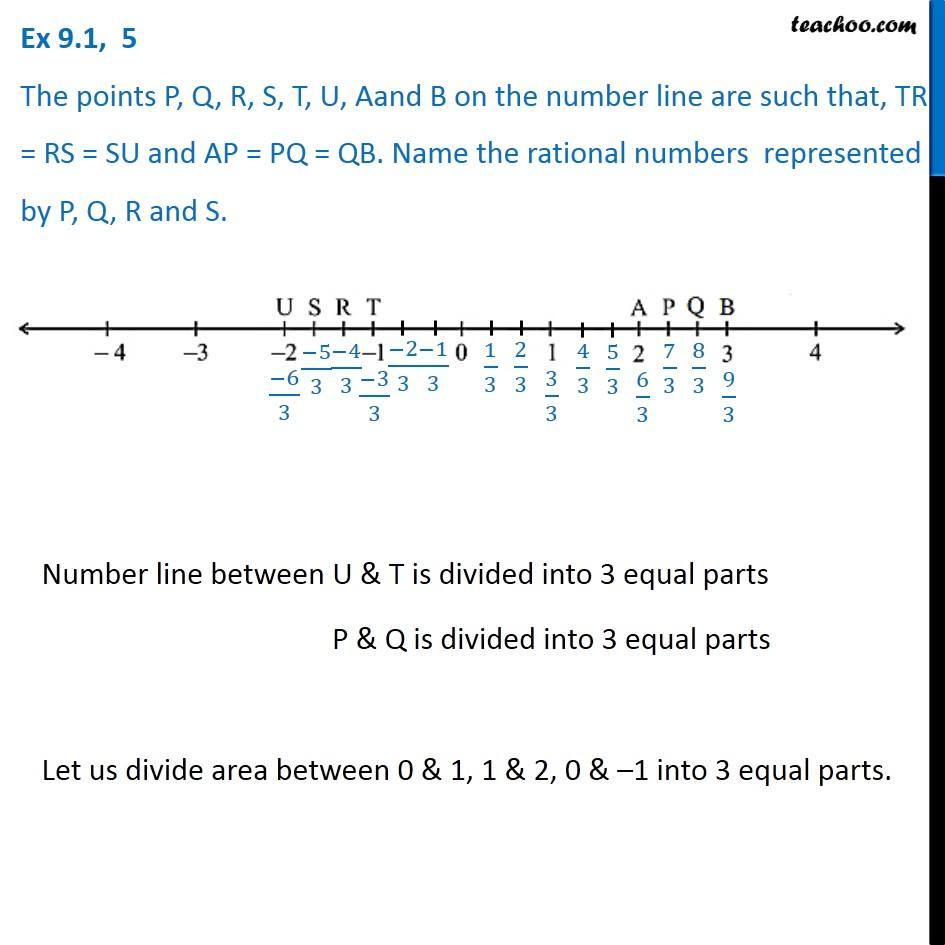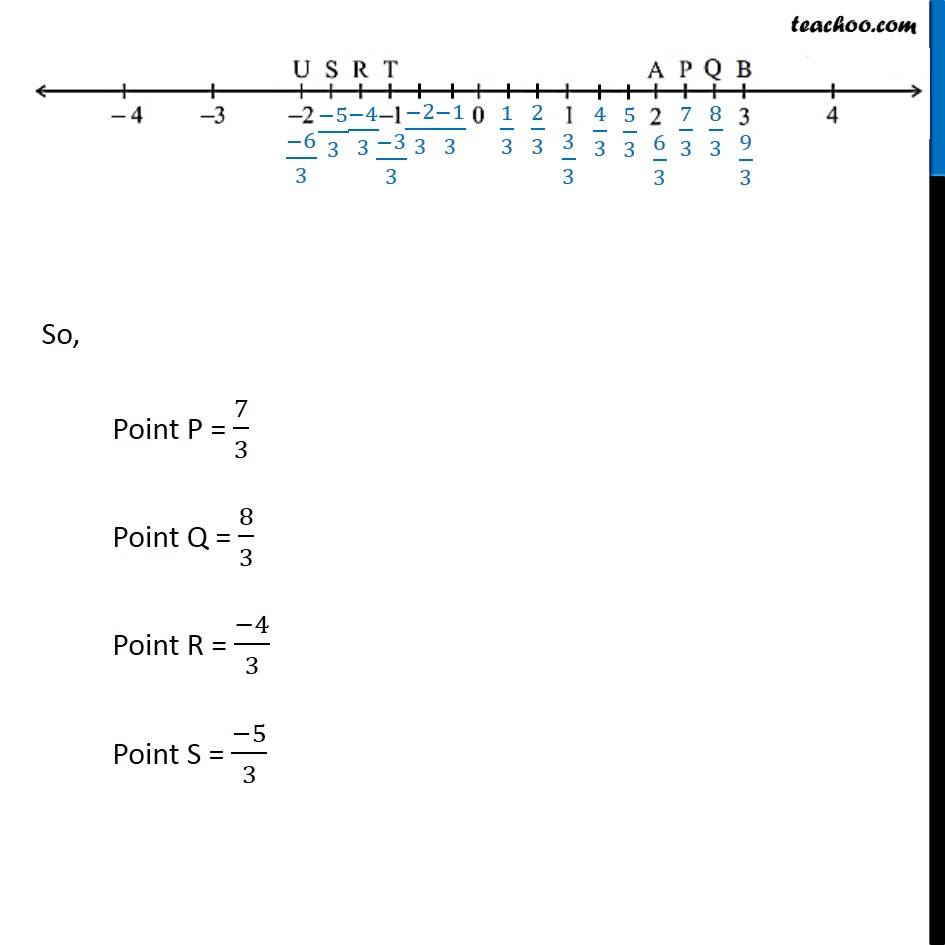Rational numbers on number line

Chapter 9 Class 7 Rational Numbers
Concept wiseIntroducing your new favourite teacher - Teachoo Black, at only ₹83 per month

### Transcript

Ex 9.1, 5 The points P, Q, R, S, T, U, Aand B on the number line are such that, TR = RS = SU and AP = PQ = QB. Name the rational numbers represented by P, Q, R and S. Number line between U & T is divided into 3 equal parts P & Q is divided into 3 equal parts Let us divide area between 0 & 1, 1 & 2, 0 & –1 into 3 equal parts. So, Point P = 7/3 Point Q = 8/3 Point R = (−4)/3 Point S = (−5)/3